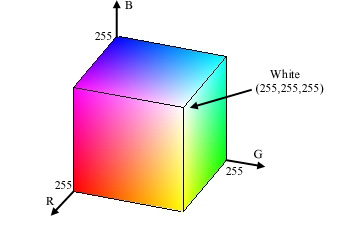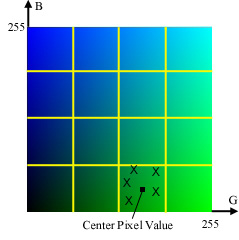Image Processing Toolbox User's GuideColor Approximation

To reduce the number of colors in an image, use the `rgb2ind` function. This function converts a truecolor image to an indexed image, reducing the number of colors in the process. `rgb2ind` provides the following methods for approximating the colors in the original image:

• Quantization
• Uniform quantization
• Minimum variance quantization
• Colormap mapping

The quality of the resulting image depends on the approximation method you use, the range of colors in the input image, and whether or not you use dithering. Note that different methods work better for different images. See Dithering for a description of dithering and how to enable or disable it.

Quantization

Reducing the number of colors in an image involves quantization. The function `rgb2ind` uses quantization as part of its color reduction algorithm. `rgb2ind` supports two quantization methods: uniform quantization and minimum variance quantization.

An important term in discussions of image quantization is RGB color cube, which is used frequently throughout this section. The RGB color cube is a three-dimensional array of all of the colors that are defined for a particular data type. Since RGB images in MATLAB can be of type `uint8`, `uint16`, or `double`, three possible color cube definitions exist. For example, if an RGB image is of class `uint8`, 256 values are defined for each color plane (red, blue, and green), and, in total, there will be 224 (or 16,777,216) colors defined by the color cube. This color cube is the same for all `uint8` RGB images, regardless of which colors they actually use.

The `uint8`, `uint16`, and `double` color cubes all have the same range of colors. In other words, the brightest red in a `uint8` RGB image appears the same as the brightest red in a `double` RGB image. The difference is that the `double` RGB color cube has many more shades of red (and many more shades of all colors). The following figure shows an RGB color cube for a `uint8` image.RGB Color Cube for uint8 Images

Quantization involves dividing the RGB color cube into a number of smaller boxes, and then mapping all colors that fall within each box to the color value at the center of that box.

Uniform quantization and minimum variance quantization differ in the approach used to divide up the RGB color cube. With uniform quantization, the color cube is cut up into equal-sized boxes (smaller cubes). With minimum variance quantization, the color cube is cut up into boxes (not necessarily cubes) of different sizes; the sizes of the boxes depend on how the colors are distributed in the image.

Uniform Quantization.   To perform uniform quantization, call `rgb2ind` and specify a tolerance. The tolerance determines the size of the cube-shaped boxes into which the RGB color cube is divided. The allowable range for a tolerance setting is [0,1]. For example, if you specify a tolerance of `0.1`, the edges of the boxes are one-tenth the length of the RGB color cube and the maximum total number of boxes is

• ``````n = (floor(1/tol)+1)^3
``````

The commands below perform uniform quantization with a tolerance of 0.1.

• ```RGB = imread('peppers.png');
[x,map] = rgb2ind(RGB, 0.1);
```

The following figure illustrates uniform quantization of a `uint8` image. For clarity, the figure shows a two-dimensional slice (or color plane) from the color cube where red=0 and green and blue range from 0 to 255. The actual pixel values are denoted by the centers of the x's.Uniform Quantization on a Slice of the RGB Color Cube

After the color cube has been divided, all empty boxes are thrown out. Therefore, only one of the boxes is used to produce a color for the colormap. As shown earlier, the maximum length of a colormap created by uniform quantization can be predicted, but the colormap can be smaller than the prediction because `rgb2ind` removes any colors that do not appear in the input image.

Minimum Variance Quantization.   To perform minimum variance quantization, call `rgb2ind` and specify the maximum number of colors in the output image's colormap. The number you specify determines the number of boxes into which the RGB color cube is divided. These commands use minimum variance quantization to create an indexed image with 185 colors.

• ```RGB = imread('peppers.png');
[X,map] = rgb2ind(RGB,185);
```

Minimum variance quantization works by associating pixels into groups based on the variance between their pixel values. For example, a set of blue pixels might be grouped together because they have a small variance from the center pixel of the group.

In minimum variance quantization, the boxes that divide the color cube vary in size, and do not necessarily fill the color cube. If some areas of the color cube do not have pixels, there are no boxes in these areas.

While you set the number of boxes, `n`, to be used by `rgb2ind`, the placement is determined by the algorithm as it analyzes the color data in your image. Once the image is divided into `n` optimally located boxes, the pixels within each box are mapped to the pixel value at the center of the box, as in uniform quantization.

The resulting colormap usually has the number of entries you specify. This is because the color cube is divided so that each region contains at least one color that appears in the input image. If the input image uses fewer colors than the number you specify, the output colormap will have fewer than `n` colors, and the output image will contain all of the colors of the input image.

The following figure shows the same two-dimensional slice of the color cube as shown in the preceding figure (demonstrating uniform quantization). Eleven boxes have been created using minimum variance quantization.Minimum Variance Quantization on a Slice of the RGB Color Cube

For a given number of colors, minimum variance quantization produces better results than uniform quantization, because it takes into account the actual data. Minimum variance quantization allocates more of the colormap entries to colors that appear frequently in the input image. It allocates fewer entries to colors that appear infrequently. As a result, the accuracy of the colors is higher than with uniform quantization. For example, if the input image has many shades of green and few shades of red, there will be more greens than reds in the output colormap. Note that the computation for minimum variance quantization takes longer than that for uniform quantization.

Colormap Mapping

If you specify an actual colormap to use, `rgb2ind` uses colormap mapping (instead of quantization) to find the colors in the specified colormap that best match the colors in the RGB image. This method is useful if you need to create images that use a fixed colormap. For example, if you want to display multiple indexed images on an 8-bit display, you can avoid color problems by mapping them all to the same colormap. Colormap mapping produces a good approximation if the specified colormap has similar colors to those in the RGB image. If the colormap does not have similar colors to those in the RGB image, this method produces poor results.

This example illustrates mapping two images to the same colormap. The colormap used for the two images is created on the fly using the MATLAB function `colorcube`, which creates an RGB colormap containing the number of colors that you specify. (`colorcube` always creates the same colormap for a given number of colors.) Because the colormap includes colors all throughout the RGB color cube, the output images can reasonably approximate the input images.

• ```RGB1 = imread('autumn.tif');
 Note    The function `subimage` is also helpful for displaying multiple indexed images. For more information, see Displaying Multiple Images in the Same Figure or the reference page for `subimage`.Reducing the Number of Colors in an Image Reducing Colors in an Indexed Image